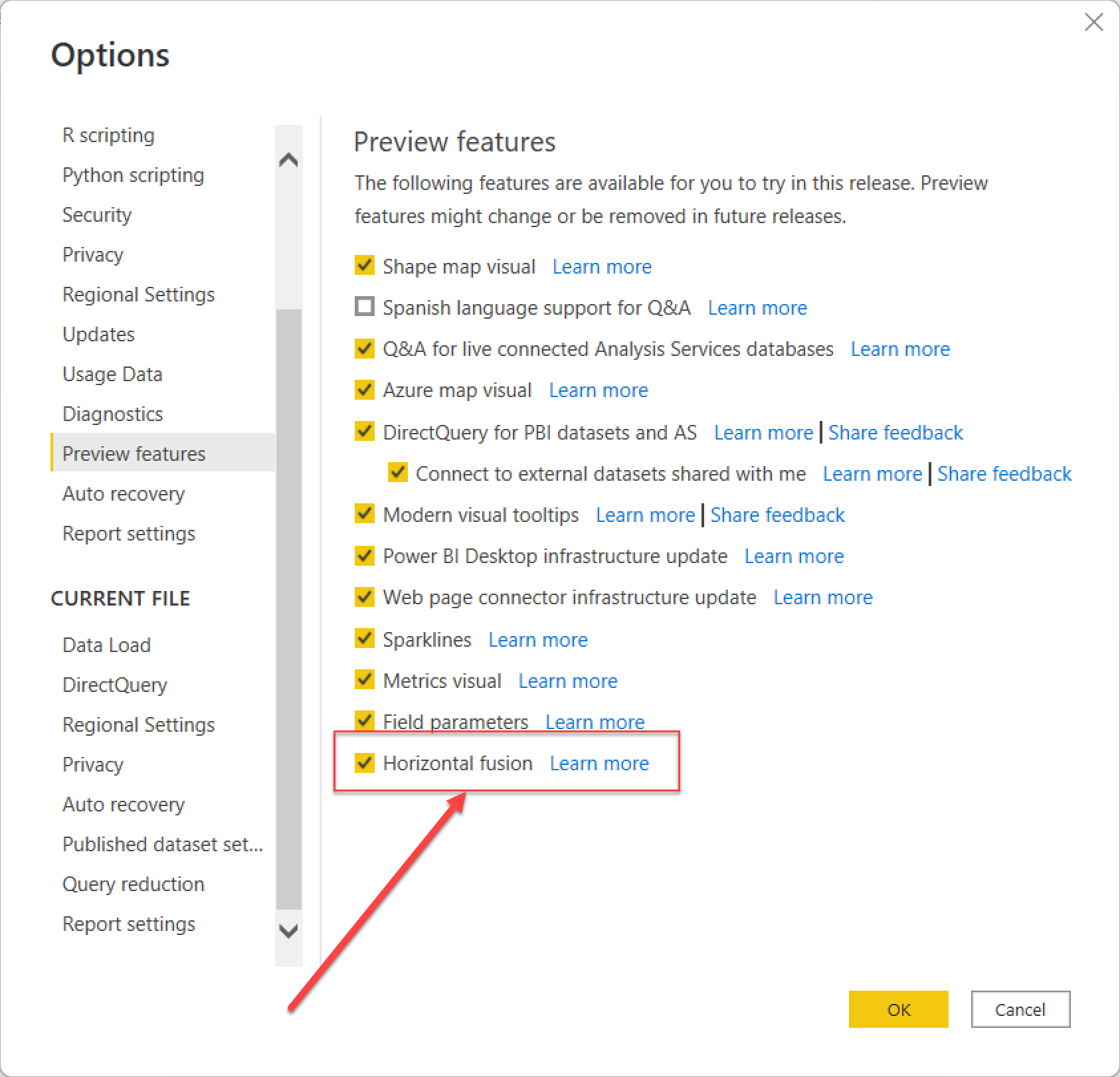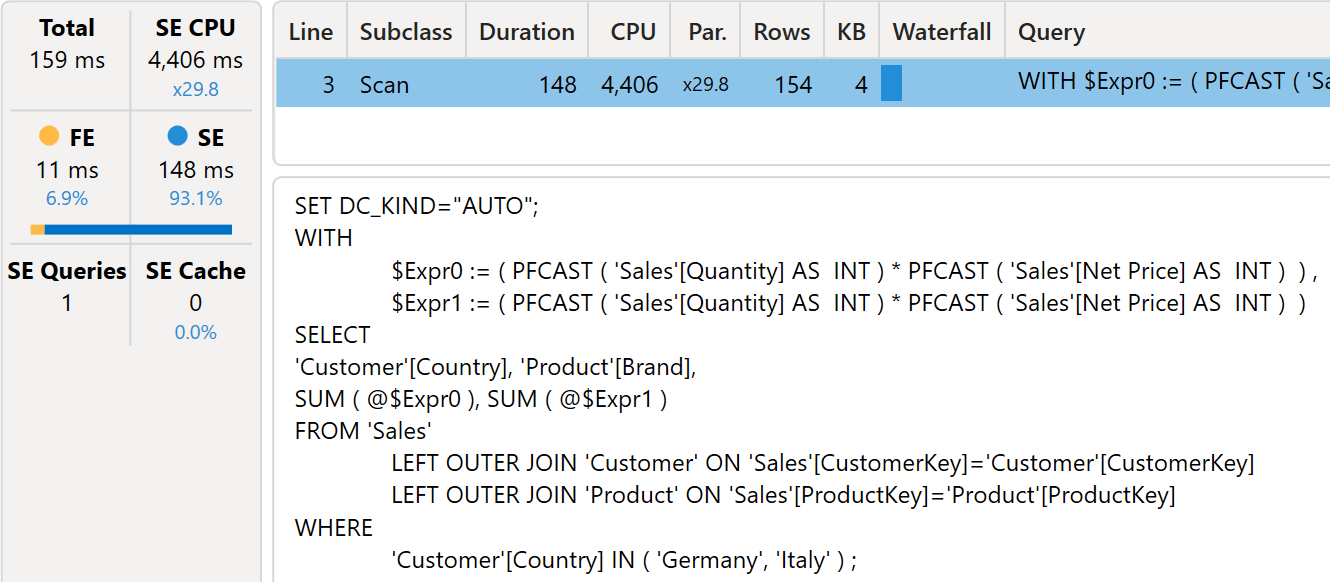Fusion is a DAX optimization that reduces the number of storage engine queries when the engine detects that multiple calculations can be merged together in a single query. There are two types of fusions: vertical fusion and horizontal fusion.

Vertical fusion occurs when multiple measures – or calculations in general – need to be computed in the same filter context. For example, the following query requires the calculation of two measures: Sales Amount and Margin:

```EVALUATE
SUMMARIZECOLUMNS (
'Product'[Brand],
"Sales Amount", [Sales Amount],
"Margin", [Margin]
)
```

Instead of executing two storage engine queries, one per measure, DAX executes a single storage engine query with both calculations being pushed down to the storage engine. Indeed, the previous query is resolved by a single xmSQL query:

```WITH
\$Expr0 :=
( PFCAST ( 'Sales'[Quantity] AS  INT ) * PFCAST ( 'Sales'[Unit Cost] AS  INT )  ),
\$Expr1 :=
( PFCAST ( 'Sales'[Quantity] AS  INT ) * PFCAST ( 'Sales'[Net Price] AS  INT )  )
SELECT
'Product'[Brand],
SUM ( @\$Expr0 ),
SUM ( @\$Expr1 )
FROM 'Sales'
LEFT OUTER JOIN 'Product'
ON 'Sales'[ProductKey]='Product'[ProductKey];
```

Vertical fusion was already available in DAX a long time ago. In the September 2022 version of Power BI however, Microsoft introduced a different type of fusion optimization: horizontal fusion.

If at the time of reading this, horizontal fusion is still in preview, you should enable it manually.Horizontal fusion aims to reduce the number of storage engine queries when similar calculations differ only by the filters applied to a column in the filter context. For example, look at the following query:

```EVALUATE
SUMMARIZECOLUMNS (
'Product'[Brand],
"Sales Amount",
CALCULATE (
[Sales Amount],
Customer[Country] = "Germany"
)
+
CALCULATE (
[Sales Amount],
Customer[Country] = "Italy"
)
)
```

In this scenario, the same measure is computed in two different filter contexts, and then the partial results of Germany and Italy are summed to produce the result. In previous versions of Power BI, the presence of two different filter contexts for the two calculations required the execution of two storage engine queries: one for Italy and one for Germany.

You can see in the following picture that there are two VertiPaq scans. The highlighted scan contains a filter for Italy.Because the two calculations differ only for the value of the Customer[Country] column being filtered, it would be possible to reduce the storage engine queries to just one. You would group by Customer[Country], so that a single query produces the result for Italy and Germany at the same time.

This is exactly what happens when horizontal fusion kicks in. The following figure shows the very same query executed with horizontal fusion enabled.There are several details to consider about the differences:

• There is a single storage engine query, compared with the previous two storage engine queries.
• The xmSQL code is grouping by Customer[Country], using Germany and Italy as a filter.
• The code runs much faster: 4,406 milliseconds of SE CPU against 5,485 milliseconds. A 20% improvement is awesome.
• Fusion does not happen at the measure level, it happens with subexpressions of the same measure.

There are multiple scenarios where horizontal fusion can greatly improve performance. Indeed, combining multiple measures that require multiple scans of the same table with slightly different filters is frequent.

Moreover, depending on the storage engine you are using, the benefit can be even larger. As you may know, VertiPaq is a very fast storage engine. If you are using DirectQuery over SQL, it is likely that your code will benefit even further. There is a huge difference between executing one query or more than one query against SQL Server, therefore we expect DirectQuery users to get more marginal improvements thanks to horizontal fusion.

In order for fusion to kick in, it is not necessary that the two calculations share the very same measure. For example, the following query computes two calculations (Sales Amount and Total Cost), with the same structure. We added the measure definition in the query to quickly spot the small differences:

```DEFINE
MEASURE Sales[Total Cost] =
SUMX (
Sales,
Sales[Quantity] * Sales[Unit Cost]
)
MEASURE Sales[Sales Amount] =
SUMX (
Sales,
Sales[Quantity] * Sales[Net Price]
)

EVALUATE
SUMMARIZECOLUMNS (
'Product'[Brand],
"Sales Amount",
CALCULATE (
[Sales Amount],
Customer[Country] = "Germany"
)
+
CALCULATE (
[Total Cost],
Customer[Country] = "France"
)
)
```

Despite the calculation not being identical, horizontal fusion works nicely, producing a single storage engine query:

```WITH
\$Expr0 :=
( PFCAST ( 'Sales'[Quantity] AS  INT ) * PFCAST ( 'Sales'[Net Price] AS  INT )  ) ,
\$Expr1 :=
( PFCAST ( 'Sales'[Quantity] AS  INT ) * PFCAST ( 'Sales'[Unit Cost] AS  INT )  )
SELECT
'Customer'[Country],
'Product'[Brand],
SUM ( @\$Expr0 ),
SUM ( @\$Expr1 )
FROM 'Sales'
LEFT OUTER JOIN 'Customer'
ON 'Sales'[CustomerKey]='Customer'[CustomerKey]
LEFT OUTER JOIN 'Product'
ON 'Sales'[ProductKey]='Product'[ProductKey]
WHERE
'Customer'[Country] IN ( 'Germany', 'France' ) ;
```

Horizontal fusion works if the different scans can be grouped in the same query. For example, grouping MIN and AVERAGE still produces a single query, because both aggregations can be computed during a single scan:

```EVALUATE
SUMMARIZECOLUMNS (
'Product'[Brand],
"Sales Amount",
CALCULATE (
MIN ( Sales[Net Price] ),
Customer[Country] = "Germany"
)
+
CALCULATE (
AVERAGE ( Sales[Quantity] ),
Customer[Country] = "France"
)
)
```

Since the average is computed by using a SUM and a count of blanks, this is the resulting xmSQL query:

```SELECT
'Customer'[Country],
'Product'[Brand],
MIN ( 'Sales'[Net Price] ),
SUM ( 'Sales'[Quantity] ),
SUM (  ( PFDATAID ( 'Sales'[Quantity] ) <> 2 )  )
FROM 'Sales'
LEFT OUTER JOIN 'Customer'
ON 'Sales'[CustomerKey]='Customer'[CustomerKey]
LEFT OUTER JOIN 'Product'
ON 'Sales'[ProductKey]='Product'[ProductKey]
WHERE
'Customer'[Country] IN ( 'Germany', 'France' ) ;
```

Mixing different aggregations (like a DISTINCTCOUNT) stops horizontal fusion from working, as the shape of the storage engine query would be too different.

Finally, horizontal fusion works if the different calculations differ for only one value in a column that can be used as a groupby column. If the filter context filters multiple values, then horizontal fusion cannot be used. The following query still generates two separate xmSQL queries, because the filter in the first calculation contains two values:

```EVALUATE
SUMMARIZECOLUMNS (
'Product'[Brand],
"Sales Amount",
CALCULATE (
[Sales Amount],
Customer[Country] IN { "Germany", "Italy" }
)
+
CALCULATE (
[Sales Amount],
Customer[Country] = "France"
)
)
```

This might be a permanent limitation, or just something to improve in the future. Check your specific scenario if you need such optimization, and verify the engine’s behavior when you test it in case it improves in the meantime.

Developers will face another limitation in case there are multiple columns that have different values in the filter context. For example, the following query could benefit from horizontal fusion, but it does not:

```EVALUATE
SUMMARIZECOLUMNS (
'Product'[Brand],
"Sales Amount",
CALCULATE (
[Sales Amount],
Customer[Country] = "Germany",
Product[Category] = "Computers"
)
+
CALCULATE (
[Sales Amount],
Customer[Country] = "France",
Product[Category] = "Audio"
)
)
```

The engine would need to produce a datacache with four values instead of only two, or it should generate an arbitrarily shaped filter. Again, we do not know if this optimization will be improved in the future.

Horizontal fusion is a great optimization. We expect many data models to benefit from the presence of horizontal fusion from day one. It does not require particular attention to the code and can improve performance of a model by a great deal.

There are multiple optimization techniques in DAX that require a thorough understanding of how the engine works. Horizontal fusion just works as is.

MINReturns the smallest value in a column, or the smaller value between two scalar expressions. Ignores logical values. Strings are compared according to alphabetical order.

`MIN ( <ColumnNameOrScalar1> [, <Scalar2>] )`

AVERAGEReturns the average (arithmetic mean) of all the numbers in a column.

`AVERAGE ( <ColumnName> )`

SUMAdds all the numbers in a column.

`SUM ( <ColumnName> )`

DISTINCTCOUNTCounts the number of distinct values in a column.

`DISTINCTCOUNT ( <ColumnName> )`